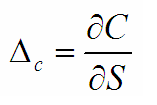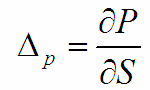# Option Greeks: Delta

Delta is the change in option premium expected from a small change in the stock price.

It is an important by-product of the Black-Scholes model.

There are three common uses of delta.

• Measure of option sensitivity
• Hedge ratio
• Likelihood of becoming in-the-money

Measure of Option Sensitivity

For a call option, the delta is represented as:For a put option, the delta is represented as:Delta indicates how many shares of stock are required to replicate the returns of the option. For example, assume that a call option has a delta of 0.75. If the stock price rises by \$1.00, the call option will increase by about 75 cents.

Delta is always positive (between 0 and 1) for long calls and short puts and negative (between 0 and -1) for long puts and short calls. For a European option, the absolute values of the put and call deltas will sum to 1. In the Black-Scholes model, the call delta is exactly equal to N(d1).

• The delta of an at-the-money option declines linearly over time and approaches 0.50 at expiration

• The delta of an out-of-the-money option approaches zero as time passes

• The delta of an in-the-money option approaches 1.0 as time passes

Hedge Ratio

Delta is also used a measure of the hedge ratio.

Assume a short option position has a delta of 0.50. If an investor owns 100 shares of the stock, he can create a perfect hedge by writing 2 calls. This is because the underlying stock always has a delta of one.

Similarly, if a portfolio of options in a stock  is +2.50, the trader would be able to hedge the portfolio by selling short 250 shares of the underlying. This is called delta-hedging.

Likelihood of Becoming In-the-Money

Some traders also use delta is a measure of the probability that an option will be in the money at expiration.

For example, if an out-of the-money option has a delta 0.20, it indicates that the option has a 20% chance of being in the money at expiry, that is the stock price will be above the option striking price at expiration.